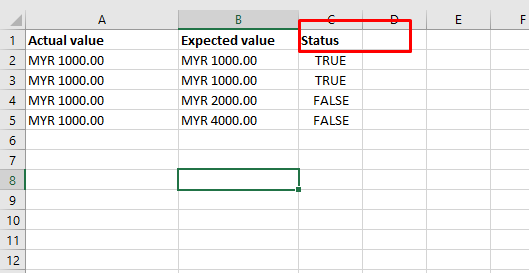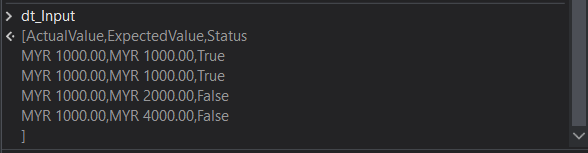# Compare two column values in the same excel and it should return a output value

In Excel, I have two columns with the same type of values and I need to compare the values and it should return an output value based on the comparison.

Ex:I have columns A and Column B with the string data type, by comparing the values I need to get the status either “True” or “False”.

use this Linq

`dt.AsEnumerable.ToList.ForEach(Sub(row) row("Status") = If(row("Actual value").ToString.Equals(row("Expected Value").ToString), "True", "False"))`

Welcome to the community

you can use excel formula

`"=IF(A2=B2,""TRUE"",""FALSE"")"`

Then use auto fill range

if you want to do in datatable then use as below in invoke code

pass dt as in/out argument with datatable type

`dt.AsEnumerable.ToList.ForEach(sub(r) r("Status") = If(r(0).ToString.Trim.ToLower.Equals(r(1).ToString.Trim.ToLower),"TRUE","FALSE"))`

cheers

I Tried like below. But the control always going

to else condition.

you also try in this way

hope this helps

you need to pass like this

CurrentRow(“ActualAmountPayable”).tostring.trim.equals(CurrentRow(“Manual Calculation”).tostring.trim)

or

CurrentRow(“ActualAmountPayable”).tostring.trim=CurrentRow(“Manual Calculation”).tostring.trim

Try with `currentRow("Column1").ToString.Trim.Tolower.Equals(currentRow("Column2").ToString.ToLower.Trim)`

but one issue with your aaproach is how do you change the row values?

if you use auto increment it will not work as expected as two separate activities of write cell are used

please try the above specified approaches

cheers

Cheers!!

You can try this in Invoke Code

``````For Each r As DataRow In in_dt.AsEnumerable()
r("Status")=If(r("ActualValue").ToString.Equals(r("ExpectedValue").ToString),True,False)
next r
``````

Output: -